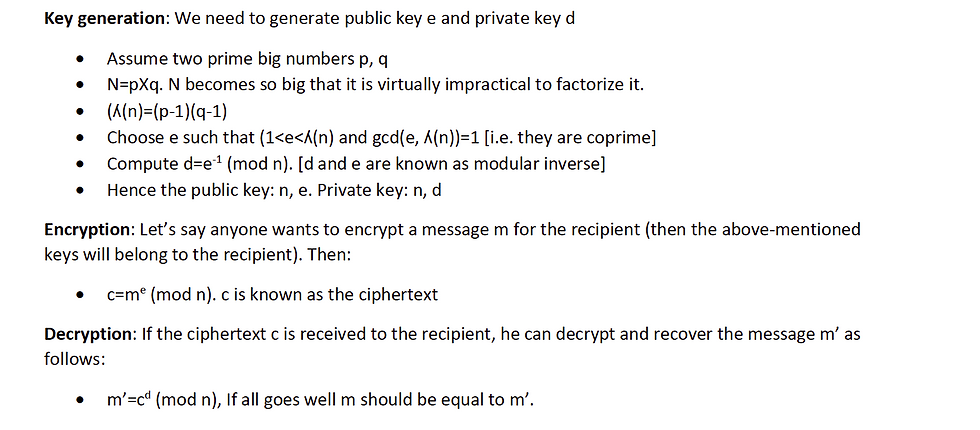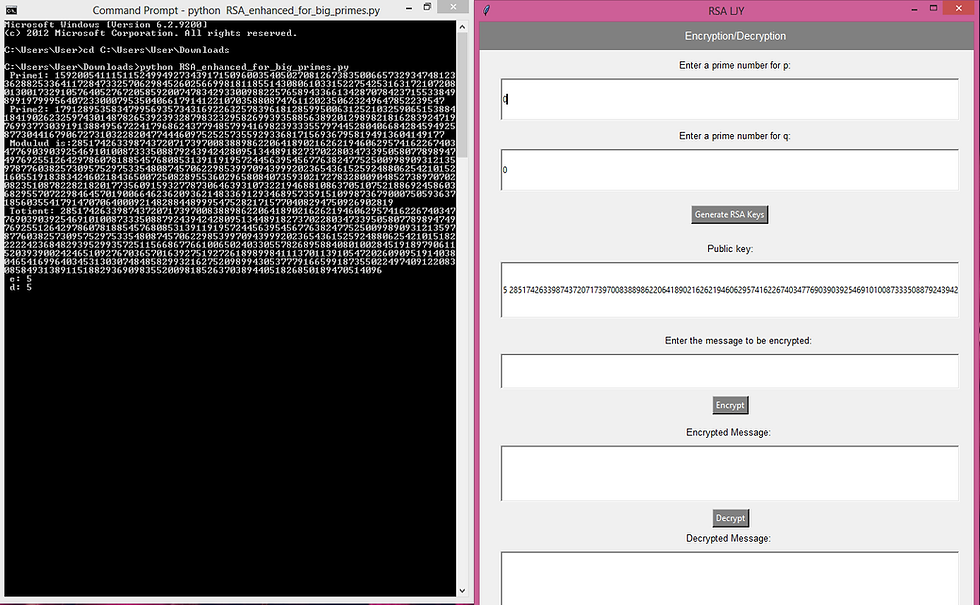top of page
Search

# RSA implementation from your own using GUI

## ICT582 Major Assignment 2020

This assignment aims to provide a Python solution to a specific implementation problem. To solve this problem, you need the knowledge gained from various Topics of this Unit. You may also need to undertake independent research to find out some Python packages, modules and functions required in your solution.### Description of the Problem

RSA implementation from your own using GUI

You can start learning GUI from: https://www.youtube.com/watch?v=J-chyaIVuzE . You are also encouraged to learn more detail (as much as you need for your assignment) about graphics libraries e.g. tkinter.

RSA is one of the most popular public key encryption algorithms which is used all over the world in most applications. The RSA algorithm involves various mathematical operations that make the encryption happen. There are three major operations involved in RSA namely: Key generation, encryption and decryption. Some of the following tutorials would explain RSA in more detail. The unit lecturer will also explain about it in one of the lectures.

The mathematical summarization of the RSA operations (key generations, encryption and decryption) are as follows:

Key generation: We need to generate public key e and private key d

• Assume two prime big numbers p, q

• N=pXq. N becomes so big that it is virtually impractical to factorize it.

• (ʎ(n)=(p-1)(q-1)

• Choose e such that (1<e<ʎ(n) and gcd(e, ʎ(n))=1 [i.e. they are coprime]

• Compute d=e-1 (mod n). [d and e are known as modular inverse]

• Hence the public key: n, e. Private key: n, d

Encryption: Let’s say anyone wants to encrypt a message m for the recipient (then the above-mentioned keys will belong to the recipient). Then:

• c=me (mod n). c is known as the ciphertext

Decryption: If the ciphertext c is received to the recipient, he can decrypt and recover the message m’ as follows:

• m’=cd (mod n), If all goes well m should be equal to m’.

An example:

Key Generation

➢ p=11, q=3, n=33

➢ ʎ(n)=10.2=20 (e.g. the Totient function λ(p) = φ(p) = p − 1=10)

➢ Choose e=3. Check gcd(e, ʎ(n))=1

➢ Try for d so that d.e (mod 20)=1. e.g. d=7

➢ Public Key: (n, e)=(33,3), Private Key: (n, d)=(33,7)

Encryption:

➢ m=7, c=73 (mod 33)=343 mod 33=13

Decryption:

➢ m’=137 (mod 33)=7 [even this works if d=27]

Write a GUI based Python program that will allow the user to perform (i) generate RSA keys, (ii) encrypt a given message and (iii) decrypt the message without using any cryptographic library. Your program will generate the values of p unless the user provides them manually. Then the program will generate the keys (private and public). Then the program will allow the user to enter his/her message to be encrypted. Finally, the program will perform the decryption operation as well. Following link may give you some more ideas about the expectation of your program.

https://www.mobilefish.com/services/rsa_key_generation/rsa_key_generation.php

• Friendly and logical GUI

• Key generation

• Encryption

• Decryption

• Performance enhancement : Due to the limitation of the size of integer type variables, many operations such as xy, xy etc. would not be possible when the value of x, y will be very high. Hence, you would not be able to work with big prime numbers. By definition, this will provide a weak RSA. Under this task you have to optimize the algorithm and implementation to support bigger number. You have to include an extra section in the report to explain how you have achieved this enhancement.

• Report and presentation

Solution Screenshots:Contact Us to get complete GUI solution at affordable price at: contact@codersarts.com